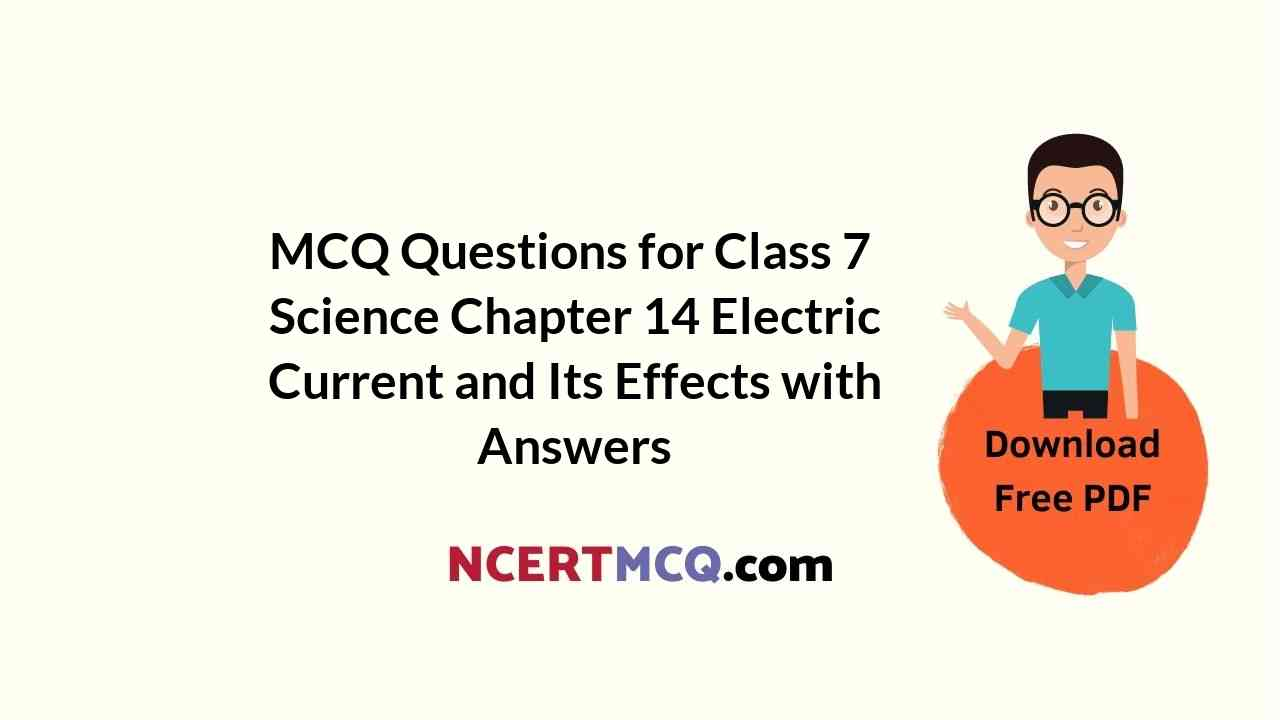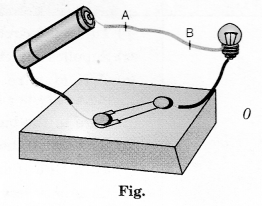Check the below NCERT MCQ Questions for Class 7 Science Chapter 14 Electric Current and Its Effects with Answers Pdf free download. MCQ Questions for Class 7 Science with Answers were prepared based on the latest exam pattern. We have Provided Electric Current and Its Effects Class 7 Science MCQs Questions with Answers to help students understand the concept very well. https://ncertmcq.com/mcq-questions-for-class-7-science-with-answers/

You can refer to NCERT Solutions for Class 7 Science Chapter 14 Electric Current and Its Effects to revise the concepts in the syllabus effectively and improve your chances of securing high marks in your board exams.

## Electric Current and Its Effects Class 7 MCQs Questions with Answers

Electric Current And Its Effects Class 7 MCQ Question 1.
In making a battery
(a) positive terminal of one cell is connected to the negative terminal of the next cell
(b) positive terminal of one cell is connected to the positive terminal of the next cell
(c) negative terminal of one cell is connected to the negative terminal of the next cell
(d) none of the above

Answer: (a) positive terminal of one cell is connected to the negative terminal of the next cell

Class 7 Science Chapter 14 MCQ Question 2.
Where can the key or switch be placed in the circuit?
(a) Left side of the battery
(b) Right side of the battery
(c) Can be placed anywhere in the circuit
(d) Near the positive terminal of the bulb

Answer: (c) Can be placed anywhere in the circuit

Class 7 Electric Current And Its Effects MCQ Question 3.
Which one of the following is based on the heating effect of current?
(a) Geyser
(b) Hair dryer
(c) Immersion rod
(d) All of these

Electric Current And Its Effects Class 7 MCQ With Answers Question 4.
The coil of wire contained in an electric heater is known as
(a) component
(b) element
(c) circuit
(d) spring

MCQ On Electric Current And Its Effects Class 7 Question 5.
The amount of heat produced in a wire depends on
(a) material
(b) length
(c) thickness
(d) all of these

Ncert Class 7 Science Chapter 14 MCQ Question 6.
Which mark is necessary on electric appliances?
(a) AGMARK
(b) ISI
(c) FICCI
(d) KSK

Class 7 Science Ch 14 MCQ Question 7.
When an electric current flows through a copper wire AB as shown in Figure, the wire(a) deflects a magnetic needle placed near it
(b) becomes red hot
(c) gives electric shock
(d) behaves like a fuse

Answer: (a) deflects a magnetic needle placed near it

MCQ Questions For Class 7 Science Chapter 14 Question 8.
Choose the statement which is not correct in the case of an electric fuse.
(a) Fuses are inserted in electric circuits of all buildings.
(b) There is a maximum limit on the current which can safely flow through the electric circuits.
(c) There is a minimum limit on the current which can safely flow in the electric circuits.
(d) If a proper fuse is inserted in a circuit it will blow off if current exceeds the safe limit.

Answer: (c) There is a minimum limit on the current which can safely flow in the electric circuits.

Electricity Class 7 MCQ With Answers Question 9.
When a switch is in OFF position.
(i) circuit starting from the positive terminal of the cell stops at the switch.
(ii) circuit is open.
(iii) no current flows through it.
(iv) current flows after some time.
Choose the combination of correct answer from the following:
(a) all are correct
(b) (ii) and (iii) are correct
(c) only (iv) is correct
(d) only (i) and (ii) are correct

Answer: (b) (ii) and (iii) are correct

Electric Current And Its Effects MCQ Question 10.
Which of the following precautions need not be taken while using electric gadgets / appliances/circuit?
(a) We should never touch a lighted electric bulb connected to the mains.
(b) We should never experiment with the electric supply from the mains or a generator or an inverter.
(c) We should never use just any wire or strip of metal in place of a fuse.
(d) We should never turn the switch in ON position.

Answer: (d) We should never turn the switch in ON position.

Match the following:

 Column A Column B (i) Switch (a) Coil of wire which heats up when electricity current is supplied (ii) Battery (b) Blows off, if the current exceeds safe limit (iii) Element (c) Consumes less energy than a bulb (iv) Filament (d) Mark that ensures that the electric appliance is safe to handle (v) Fuse (e) Supplies current to the circuit (vi) MCBs (f) Turns the circuit ON and OFF (vii) CFL (g) Turn OFF if current exceeds safe limit (viii) ISI (h) Wire in the bulb which glows

 Column A Column B (i) Switch (f) Turns the circuit ON and OFF (ii) Battery (e) Supplies current to the circuit (iii) Element (a) Coil of wire which heats up when electricity current is supplied (iv) Filament (h) Wire in the bulb which glows (v) Fuse (b) Blows off, if the current exceeds safe limit (vi) MCBs (g) Turn OFF if current exceeds safe limit (vii) CFL (c) Consumes less energy than a bulb (viii) ISI (d) Mark that ensures that the electric appliance is safe to handle

Fill in the blanks:

1. Our body is a ………………….. of electricity.

2. An electric cell produces electricity from the ………………….. in it.

3. In an electric circuit a fuse is a ………………….. to prevent possible fire.

4. A combination of two or more cells is called a ………………….. .

5. The coil of wire in an electric heater is called an ………………….. .

6. A ………………….. is a safety device which prevents damages to electrical circuits and possible fires.

7. The wire gets ………………….. when an electric current passes through it.

8. We must look for ………………….. mark on electrical appliances.

9. When electric current passes through a wire, it behaves like a magnet. It is the ………………….. effect of current.

10. Crane has a strong ………………….. attached to it.

Choose the true and false statements from the following:

1. It is convenient to represent electric components by symbols.

2. A connecting wire is symbolized by a zig-zag line in the circuit diagram.

3. When an electric current flows through a wire, the wire gets heated.

4. The key or switch can be placed anywhere in the circuit.

5. The amount of heat produced in a wire depends on its material, length and thickness.

6. CFLs consume more electricity than ordinary bulbs.

7. For different requirements, the wires of different materials, different lengths and thicknesses are used.

8. A fuse is used to save energy in electrical circuits.

9. MCBs are the switches which automatically turn off when current in a circuit exceeds the safe limit.